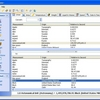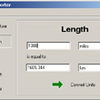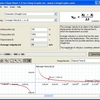# Abf value converter

ABF Value Converter is an application for measurement units conversion. This software easily converts various measurement values into any other possible ones. Inches to centimetres, pounds to kilograms, Fahrenheit to Celsius... and more than 5000 other conversions, grouped according to categories. ABF Value Converter also calculates the values of many mathematical functions, as well as makes it possible to conduct geometric constructions and calculations for some figures.

ABF Value Converter contains 11 conversion calculators (one per measurement group), 3 geometric calculators, 2 calculators of the most common functions and a converter between different number systems such as Dec, Hex, Bin and Roman. The user interface of this program is based upon opinions of different users. The Outlook-styled bar makes navigation easy and convenient. Program interface is customized, so the users can change it, according to their preferences.

## Related software (5)## Conversions+

Conversion software that converts over 600 different measurements. Linear Measure, Area, Temperature, Weight, Liquid, Volume, Speed and Time measurements can all be converted withi ...## Dicom Unit Aware Calculator

Dicom is the first ever unit aware calculator of its kind that deals with dimensions of physical quantities in mathematical expression. Dicom enables calculations, conversions, and ...## Easy Unit Converter

Easy Unit Converter converts temperature, length, area, volume, weight with a single button click. Easy Unit Converter includes about 50 most frequently used measurement units. Thi ...## Galactic Geometry 3D

Calculate volume and surface area to blast away rocks and save your space ship! Galactic Geometry is an engaging 3D environment for learning about geometric figures and their equat ...## Physics Cheat Sheet

Physics Cheat Sheet is an interactive physics package that helps students solve and visualize numerous physics equations.